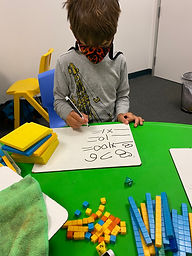## Ms. Briana

### Target 1​

###### Lesson Type:

Continuation

Number Operation

:

Computation

Subtract within 1,000 (regrouping limited to only one place-value).

###### 1:

Be introduced to a variety of computation strategies for subtracting within 1,000 and select a strategy that works best.

###### 2:

Understand how to regroup based on place-value understandings (i.e., ten ones can be composed into one ten – ten tens can be composed into one hundred).

###### 3:

Understand that the same process is utilized to subtract three or more whole numbers that is used to subtract only two numbers.

###### 4:

Understand that when adding a multi-digit number, to solve start in the lowest place-value and go left.

1st

###### Vocabulary:

Subtract, Place-Value and Multi-Digit

Activities:

Students used small number wheels to create equations to solve.

Students labeled the different palce values while creating subtraction problems.### Home Exploration

###### Guiding Questions:## Absent Students:

### Target 2

:

###### 1:

Use a number to represent base ten block quantities.

###### 2:

Use place-value understandings to create numbers within specific limits.

###### 3:

Understand how to “regroup” based on place-value understandings (i.e., ten ones can be composed into one ten – ten tens can be composed into one hundred).

###### 4:

Represent a multi-digit number in expanded format.

###### 5:

Represent the composition or decomposition of three-digit numbers with a standard expanded format equation using both multiplication and addition (384 = (3x100) +(8x10) +(4x1)).

2nd

###### Vocabulary:

Composition, Expanded Form, Standard Form and Re-Group

Activities:

Students used base 10 blocks to represent 4 digit quantities. (Students used 8 sided dice to create the 4 digit quantity)

Students used base 10 blocks to visually represnet expanded form and wrote it out on personal white boards.### Home Exploration

###### Guiding Questions:### Target 3

:

###### Vocabulary:

Activities:### Home Exploration